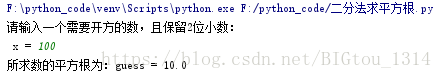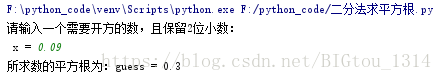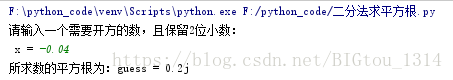# 基于Python的二分法求平方根

``````	输入：x
输出：√x
步骤：
①low = 0；high = x；
②guess = （low + high） / 2
③如果guess² == x，则输出guess，程序结束；
④如果guess² < x，则low = guess，继续执行步骤②
⑤如果guess² > x，则high = guess，继续执行步骤②
``````

``````				    if guess ** 2 < x:
low = guess

else:
high = guess

guess = (low + high) / 2
``````

``````x = float(input("请输入一个需要开方的数，且保留2位小数：\n x = "))
``````

``````				abs(guess ** 2 - x) > 0.0001
````````````					if x > 0 and x < 1:
high = 1.0
low = 0
guess = (low + high) / 2
````````````				 if x == 1.0 and x == 0.0:
guess = x
``````

``````	①取一个负数记为x_org；
②将 （-x_org）赋给x；
③x为正，带入前面的运算计算；
④输出结果后面加个字符“j”，程序结束。
``````

``````						if x_org < 0:
x = - x_org
else:
x = x_org
``````
``````			elif x_org == -1.0:
print("所求数的平方根为：guess = j" )
else:
print("所求数的平方根为：guess = %.1fj" % (guess))
``````## 重点来了：话不多说，直接上代码

``````	#!/usr/bin/python3

# 利用二分法求解平方根

x_org = float(input("请输入一个需要开方的数，且保留2位小数：\n x = "))

# 判定x_org的正负，负数取反
if x_org < 0:
x = - x_org
else:
x = x_org
# 变量的初始化
## 程序第①步
low = 0.0
high = x
## 程序第②步
guess = (low + high) / 2

if x > 0 and x < 1:
high = 1.0
low = 0
guess = (low + high) / 2

# 二分法求解平方根
if x >= 0:
while abs(guess ** 2 - x) > 0.0001:
if x > 1.0:# 大于1 的数
if guess ** 2 < x:
low = guess # 如果guess² < x，则low = guess，
else:
high = guess # 如果guess² > x，则high = guess，
guess = (low + high) / 2 # 继续执行

if x == 1.0 and x == 0.0:# 0 和1 的求解
guess = x

else:
if guess ** 2 < x: # 在0-1之间的数
low = guess
else:
high = guess
guess = (low + high) / 2

# 结果输出
if x_org >= 0:## 非负数输出
print("所求数的平方根为：guess = %.1f"%(guess))
## 负数输出
elif x_org == -1.0:
print("所求数的平方根为：guess = j" )
else:
print("所求数的平方根为：guess = %.3fj" % (guess))

``````

## 总结

``````		if x > 0 and x < 1:
n = int(input("请输入被开方的小数位数：\n n = "))
count = 10**n
x = x * count
high = x
guess = (low + high) / 2
count_high = count
count_low = 0
count_guess = (count_low + count_high) / 2

while abs(count_guess ** 2 - count) > 0.0001:
if count_guess ** 2 < count:
count_low = count_guess
else:
count_high = count_guess
count_guess = (count_low + count_high) / 2
``````
``````			if guess ** 2 < x:
low = guess
else:
high = guess
guess = (low + high) / （2*count_guess)

``````